## Journal of Chemistry and Applied Chemical EngineeringISSN : 2576-3954

All submissions of the EM system will be redirected to Online Manuscript Submission System. Authors are requested to submit articles directly to Online Manuscript Submission System of respective journal.

Research Article, J Chem Appl Chem Eng Vol: 1 Issue: 2

# Oxidation Number, Oxidant and Reductant as Derivative Concepts within GATES/GEB Formulation

Anna Maria MichaÅowska-Kaczmarczyk1, Aneta Spórna-Kucab2 and Tadeusz MichaÅowski2*

1Department of Oncology, The University Hospital in Cracow, 31-501 Cracow, Poland

2 Department of Analytical Chemistry, Technical University of Cracow, 31-155 Cracow, Poland

Faculty of Chemical Engineering and Technology, Cracow University of Technology, Warszawska 24, 31-155 Cracow, Poland
Tel: +48126282035
E-mail: [email protected]

Received: October 23, 2017 Accepted: November 11, 2017 Published: November 18, 2017

Citation: MichaÅowska-Kaczmarczyk AM, Spórna-Kucab A, MichaÅowski T (2017) Oxidation Number, Oxidant and Reductant as Derivative Concepts Within GATES/GEB Formulation. J Chem Appl Chem Eng 1:2. doi: 10.4172/2576-3954.1000109

## Abstract

Formulation of Generalized Electron Balance (GEB) according to Approach II to GEB, based on linear combination f12 = 2?f(O) – f(H) of elemental balances: f(H) for H and f(O) for O, is illustrated on the titration of NaBr + H2SO4 (as titrand, D) with Ce(SO4)2 + H2SO4 solution (as titrant, T), according to GATES/GEB principles. Some fundamental advantages inherent in linear combination of f12 with other elemental/core balances are indicated. The prior information on oxidation numbers of all elements participating the redox system is not needed. The multipliers needed to obtain the simpler form of the balance resulting from the linear combination are equal to oxidation numbers of the elements participating the related system. This way, the f12 provides the criterion distinguishing between nonredox and redox systems. The f12 is linearly independent on other balances in a redox system, or linearly dependent in non-redox systems; the D and T are considered here as non-redox subsystems of the D+T redox system. All available physicochemical knowledge related to the D+T system can be involved in the iterative computer program used for calculation purposes.

## Introduction

Redox systems are the most important/complex electrolytic systems. Redox reactions are usually coupled with acid-base, and – frequently – with complexation and/or precipitation reactions. There are redox systems with one (disproportionation, symproportionation), two or more electron-active elements. Moreover, a particular type (e.g. complexation) reaction may be exemplified by different representatives (here: ligands).

The commonly known viewpoint on titrations in electrolytic redox systems was introduced mainly in the 1960s in a series of papers by Bard and Simonsen , Bishop [2,3], followed by the articles of Goldman [4-10], and outlined later by Goldman . The viewpoint (well-being) of those authors on their own achievements in this regard, expressed (among others) in the titles of their papers, was so amazingly contagious and convincing for the next generations of chemists, that it was replicated in numerous papers and handbooks issued later, e.g. [12-14], and in learning materials, available today in the Internet. Those elaborations/viewpoints, based on stoichiometric inferences, were totally criticized/disqualified in the review paper , and in [16-20].

The correct resolution of electrolytic redox systems, made according to the principles of Generalized Approach to Electrolytic Systems (GATES) , was presented in [15-46]. When referred to redox systems, GATES, involves the Generalized Electron Balance (GEB) in the software denoted as GATES/GEB.

Within the GATES formulation, the species in an electrolytic system are considered in their natural forms, particularly as hydratesin aqueous solutions  (p. 520), where zi (zi = 0, ±1, ±2,…) is the charge of Xizi , expressed in terms of elementary charge unit e = F/NA (F – Faraday constant, NA – Avogadro’s constant); ni≡niW≡niH2O (≥ 0) is the mean number of water (W = H2O) molecules attached to Xizi . The known chemical formulas of the Xizi and their respective external charges provide the information necessary/sufficient to formulate the respective balances for elements or cores.

The terms: components and species are distinguished. In the notation applied here, N0j (j=1,2,…,J) is the number of molecules of the component of j-th kind, including water, forming the static or dynamic D+T system, from titrand D and titrant T composed separately. The mono- or two-phase electrolytic system thus obtained involves N1 molecules of H2O and Ni species of i-th kind,(i=2, 3,…,I), denoted briefly as Xizi (Ni,ni); then we have: H+1 (N2,n2), OH-1 (N3,n3),… .

The process considered is the titration, where V0 mL of titrand D is titrated with V mL of titrant T; V is the volume of T added up to a defined point of the titration; the D and T are considered as subsystems of the D+T mixture thus formed. The volume of D+T at this point of the titration is V0+V, if the assumption of additivity in volumes is admitted/tolerable. The Ni speciesin the D+T mixture are denoted as Xizi (Ni,ni), i=2,…,I, whose molar [mol/L] concentrations are denoted as [Xizi ], and(1)

Some species in a system may involve a core, i.e., a common group/cluster of elements with defined composition, structure and external charge, that is unchanged during a process realized in the system. For example, in Ce(SO4)2 (C) + H2SO4 (C1) solution, applied as a titrant T in cerimetric  titrations we have, among others, the hydrated species of: HSO4-1, SO4-2, CeSO4+2, Ce(SO4)2 and Ce(SO4)3 -2, with SO4-2 as the common core.

Balancing of electrolytic systems according to GATES/GEB principles

When balancing an electrolytic system, where K different elements/cores are involved, we apply the rules of:

(a) charge conservation, expressed by charge balance, f0 = ChB, and

(b) elements/cores conservation, termed as elemental/core balances, fk = f(Yk),

where Yk is an element or core of k-th kind (k=1,…,K); in particular, we have: f1 = f(H) for Y1= H, f2 = f(O) for Y2= O.

The numbers Ni of the species are interrelated in the charge balance, f0 = ChB,(2)

In fact, only charged species (zi≠0) enter Equation 2. The Ni and N0j are interrelated in elemental/core balances fk, written as follows(3)

In particular, we have:(4)

for H (k=1), where α1i is the number of H-atoms involved in Xizi, and(5)

for O (k=2), where α2i is the number of O-atoms involved in Xizi. Then from Equations 4, 5 we get:(6)

i.e., the numbers ni of water molecules are cancelled, at any i-value. From Equations 3, 6 we have the balance(7)

where d1j and d2j are the numbers of atoms: H and O, resp. in one molecule of the j-th component Y0j. For k=3,…,K, aki is a number of elements/cores of i-th kind in Xizi, and dkj (k=1,…,K) is a number of elements/cores of k-th kind in Y0j. Linear combination of the balances: 2, 7 and 3 for k=3,…,κ, where κ ≤ K, with pre-assumed coefficients/ multipliers mk, gives(8)(9)(10)

When the multipliers mk are equal to the oxidation numbers of elements in the corresponding balances (3) for k=3,…, κ, we get the simpler(st) forms of the linear combinations, not involving the species composed only from electron-non-active elements. Note that m1= +1 and m2= –2 in Equation 10 are the oxidation numbers for H and O in components and the species of the system in question .

It is advisable to start formulation of the system from balances interrelating the numbers N0j of particular components Yj forming V0 mL of titrand (D) and V mL of titrant (T) and the numbers Ni of particular speciesformed in the D+T mixture, at a given point of the titration.

This article refers immediately to a dynamic redox system, considered in  according to the old viewpoint, commented above. The system will be formulated here according to GATES/GEB principles.

On cerimetric titration of bromide

Referring to the example raised in , we consider V0 mL of titrand D composed of: NaBr (N01 molecules), H2SO4 (N02 molecules) and H2O (N03 molecules), titrated with V mL of titrant T composed of Ce(SO4)2â4H2O (N04 molecules), H2SO4 (N05 molecules) and H2O (N06 molecules). In V0+V mL of the D+T mixture thus formed we have the species:Note, for example, that:

N6 species of HBrO3ân6H2O, denoted for brevity as HBrO3 (N6, n6), involve: N6(1+2n6) atoms of H, N6(3+n6) atoms of O, and N6 atoms of Br;

N04 molecules of Ce(SO4)2â4H2O involve: 8N04 atoms of H, 12N04 atoms of O and O, 2N04 atoms of S, and N04 atoms of Ce.

As we see, different species are considered in their natural form, i.e. as solvates, e.g. hydrates in aqueous media. These remarks can be extended also on binary- [34,49-51] and mixed-solvent media [29,52], where formation of the species≥0) is admitted, assuming that at least one of the co-solvents As (s ∈ <1,S>) has amphiprotic properties.

Applying, for the convenience of notation, the common enumeration of the species as one presented in the set (11), we formulate the balances, separately for D, T, and D+T. We will check the properties of linear combinations of charge and elemental/core balances for each of these three solutions. Separate balancing of D and T aims to show/prove that D and T, when treated as separate solutions, are here non-redox systems.

A very useful/effective manner for checking/stating the linear dependence of the balances is the transformation of an appropriate system of equations to the identity, 0 = 0 [17,42]. To facilitate these operations, we apply the equivalent forms of the starting equationsIn this notation, f(Yk) will be essentially treated not as the algebraic expression on the left side of the equation f(Yk) = 0, but as an equation that can be expressed in alternative forms presented above. The equations in the form φ(Yk) – bk = 0 are applied in the minimization function of the computer program (Appendix).

Balancing of D

The balances for elements/species in D (NaBr + H2SO4 + H2O) and their linear combinations are as follows: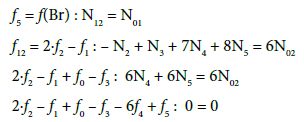Balancing of T

The balances for elements/species in T (Ce(SO4)2â4H2O + H2SO4 + H2O) and their linear combinations are as follows:Balancing of D+T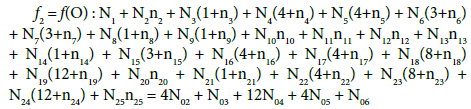(13)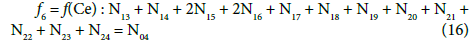On this basis we formulate the linear combinations of the balances: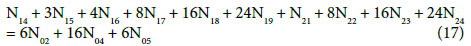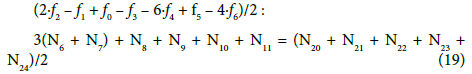Applying atomic numbers: ZBr = 35 for Br, ZCe = 58 for Ce, we get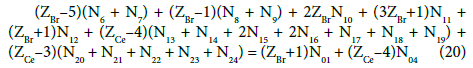Applying the relations: Equation 1 and: C0V0 = 103âN01/NA, CV = 103âN04/NA, C01V0 = 103âN02/NA, C1V = 103âN05/NA, from the balances (12) – (20) we obtain the relations expressed in terms of molar [mol/L] concentrations:[Na+1] = C0V0/(V0+V) (13a)The N01 and N04 were not involved in Equation 19 and then C0 and C are not involved in Equation 19a.The distinguishing role of f12 = 2âf(O) – f(H)

For all redox systems, any linear combination of f12 = 2âf(O) – f(H) with charge balance f0 = ChB and other elemental/core balances fk = f(Yk) (k=3,…,K) related to a redox system of any degree of complexity does not give the identity, 0 = 0 [17,41,45]. The f12 is linearly dependent on f0,f3,…,fK when related to non-redox systems. In other words, the set of K independent balances f0,f12, f3,…,fK is applied for a redox system, or a set of K–1 balances f0,f3,…,fK is applied to a non-redox system with K–2 independent elemental/core balances fk = f(Yk) for Yk ≠ H, O. The independency or dependency property of f12 is then the general criterion distinguishing between redox and non-redox systems [17,28,45] of any degree of complexity, also in mixed-solvent media [29,49,52].

The difference between redox and non-redox systems is then ‘coded’ in f12. This unique, general property explains clearly why the elemental balances: f1 and f2 are not formulated for non-redox systems, of any degree of complexity. The f12 and any linear combination of f12 with f0,f3,…,fK for a given system retain full properties of the GEB. In other words, f12 is fully compatible with other (f0,f3,…,fK) balances and enables to resolve any electrolytic (mono- or/and two-phase) redox system within GATES, and GATES/GEB in particular.

All the inferences made within GATES/GEB are based on firmly established physical, physicochemical and algebraic foundations.

A choice of the independent equations and variables

Equations (17a), (18a), (19a) and (20a), are equivalent forms of the Generalized Electron Balance (GEB). This equivalence is perceived from the viewpoint of the linear combination of f12 with the balances related to the system in question. For calculations we take one of these equations. In the computer program in Appendix, the shortest balance expressed by Equation 19a, has been chosen.

The GEB (Equation 19a) completes the set of equations composed of charge balance (12a) and concentration balances for: Br (Equation 15a), Ce (Equation 16a), S (14a) – together 5 equations. The balance 13a, involving only one species, is not considered as equation, but as an equality , as a number that can be put, as such, into Equation 12a.

The set of independent variables is arranged in the (transposed) vector x = (E,pH,pSO4,pBr,pCe3)T, where E – potential [V], pH = –log[H+1], pSO4 = –log[SO4-2], pBr = –log[Br-1], pCe3 = –log[Ce+3]. informally, E is ascribed to GEB, pH to ChB, and pSO4, pBr, pCe3 to the related concentration balances.

The number of the (independent, ‘homogeneous’) variables equals to the number of equations, 5 = 5; this ensures a unique solution of the equations related to the system in question, at the preset C0, C, C01, C1 and V0 values, and the V-value at which the calculations are realized, at defined step of the calculation procedure offered e.g. by MATLAB , and realized according to the iterative computer program presented in Appendix.

On this basis, the functional dependencies: E = E(Φ), pH = pH(Φ) and speciation curvescan be presented graphically for different species Xizi , whereis the fraction titrated; it introduces a kind of normalization (independency on V0 value) in graphical presentation of the titrimetric procedure, compare with the related plots drawn for D+T system, where FeSO4 + H2SO4 solution as D is titrated with Ce(SO4)2 + H2SO4 solution as T [25,30].

## The Approach II to GEB versus the Approach I to GEB

GEB is perceived as a law of the matter conservation, as a relatively new Law of Nature [16,21,42]. From historical viewpoint, two equivalent approaches to GEB were applied. The Approach I to GEB is based on a “card game” principle, with electron-active elements as “players”, electron-non-active elements as “fans”, and electrons as “money”. This analogy is broadly and suggestively depicted in  (pp. 41-43). The transmission of electrons occurs between different species formed by players, whereas such transmission does not occur between fans (the fans’ accounts are intact). Another version of GEB is the Approach II, formulated on the basis of linear combination f12 = 2·f(O) − f(H), presented above.

In the D+T redox system considered here, Br and Ce are two electron-active elements, brought to the system in the salts: NaBr and Ce(SO4)2 â4H2O; the elements: H, O, Na and S are considered here as ‘fans’. W=H2O (owner of fans: H and O) is neither oxidized nor reduced in this system. N01 molecules of NaBr brings into the system (ZBr+1)N01 bromine electrons, whereas N04 molecules of Ce(SO4)2â4H2O brings here (ZCe–4)N04 cerium electrons. The common pool of electrons brought here equals (ZBr+1)N01 + (ZCe–4)N04. A part of these electrons form new bromine species and new cerium species. It is involved with transmission of electrons as “money” between Br and Ce as “players”. W=H2O accompanies formation of oxidized forms of Br, e.g. Br-1 + 3H2O – 6e-1 = HBrO3 + 5H+1. In this reaction, O does not change its oxidation number, that is equal –2 both in H1O and in BrO3-1. Likewise, H does not change its oxidation number; it is equal +1 in H2O and in H+1. So, on a particular stage of D+T formation, N6 entities of HBrO3ân6H2O as the species formed in this reaction contains (ZBr – 5)N6 bromine electrons, … , N24 entities of Ce(SO4)3-3ân24H2O contains (ZCe – 3)N24 cerium electrons (see the set (2) and Equations 15, 16). Applying the summation similar to that shown in  (p. 22), we obtain Equation 20, and then Equation 20a. Knowing that in a system of compatible equations , the equation obtained by any linear combination of a given equation with other equations are equivalent to one another; we can thus conclude that the Approach I to GEB is equivalent to the Approach II to GEB .

GEB, charge and concentration balances, together with the set of independent equilibrium constants, provide the numerical algorithm, implemented to software packages that support advanced programming, such as MATLAB computing environment. The calculation procedure enables the desired relationships to be plotted in 2D or 3D space .

In the version presented above, the GEB applies to systems where no radioactive transformations occur, and the composition of a matter reflects the natural isotopic composition of individual elements. The elements preservation principle is referred to stable elements. However, an extension of GATES on some systems where nuclear (α, β-, β+, or electron capture) transformations of radioactive elements occur is possible, assuming the known decay scheme of these elements .

## Comments on the Oxidation Numbers

The remarks resulting from the calculations presented above for D and T as the non-redox subsystems (when considered separately) of the redox D+T system (as the mixture of D and T), can be summarized as follows.

(1) Within f12 = 2âf2f1 = 2âf(O) – f(H) (Equation 11), all numbers involved with water (W=H2O), i.e., N1, N03, N06 and all ni = nW values were cancelled in D, T and D+T in aqueous media; This is an extremely advantageous situation, when perceived from the calculation viewpoint.

(2) The linear combinations: 2âf2f1 + f0f3 – 6f4 + f5 for the titrand D and 2âf2f1 + f0 – 6f4 – 4f6 for the titrant T, after multiplying by –1, can be presented in equivalent forms:are transformed into identity, 0 = 0. The appropriately selected multipliers of the corresponding balances are equal to the oxidation numbers of the elements in the corresponding species included in these balances.

(3) The linear combination 2âf2f1 + f0f3 – 6f4, involving all electron-non-active elements of the system, can be presented in equivalent forms: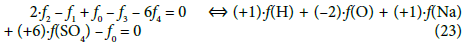This combination (Equation 18a) involves all components and species formed by the electron-active elements of the system in question; it can be rewritten into the equivalent form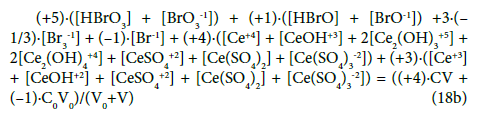In other words, all the species involved with ‘fans’ (H, O, Na and S) were cancelled within the linear combination (23). As we see, the multipliers for concentrations of the corresponding electronactive elements (Br, Ce) in the related components and species in Equation 18b are equal to oxidation numbers of these elements in the components and species. It is the exciting property from the viewpoint of the oxidation number which, after all, is seen in chemistry as the contractual concept [55-57]. It follows naturally from a proper linear combination of charge and elemental balances, which result from the basic principles of charge and elements preservation. The Approach II shows that GEB is based on a reliable law of the elements conservation, and – in this regard – it is equally robust as equations for charge and concentration balances.

On the step of formulation of the balances according to GATES/ GEB principles, the term “oxidation number” is not ascribed to any element in a complex species present in the system considered according to Approach II to GEB; in this context, it is the redundant concept only. A known composition of all the species, expressed by their formula and external charge, provides information sufficient to formulate the related balances. These facts are of capital importance when redox equilibria are involved, e.g., with complex organic species . Different species, also radicals and ion-radicals, can be included in these balances. None information about a structure of the species is needed.

The terms: oxidant and reductant are also redundant terms, not necessary (not applicable) as starting terms for further considerations on a redox system . However, the oxidation degree can easily be calculated for simple compounds and species and then the Approach I to GEB is more applicable for this purpose, as a shorter (from the viewpoint of derivation only!) form of GEB. It is the case in the example of D+T system presented in this paper.

The Generalized Electron Balance (GEB) concept, obligatory for description of redox systems and formulated by MichaÅowski as the Approach I (1992) and the Approach II (2006) to GEB, is fully compatible with charge balance (ChB) and concentration balances, and complete set of relations for the corresponding equilibrium constants.

A keystone for the overall, thermodynamic knowledge on electrolytic systems is the linear combination f12 = 2·f(O) – f(H) of elemental balances: f(H) for H and f(O) for O. The 2âf(O) – f(H) can be formulated both for non-redox and redox systems, in aqueous, non-aqueous and mixed-solvent systems, with amphiprotic (co) solvent(s) involved. The independent equation for 2·f(O) – f(H), considered as the primary form of GEB, pr-GEB = 2âf(O) – f(H), is the basis of GEB formulation according to Approach II to GEB. The 2·f(O) – f(H) is linearly independent on ChB and other balances, for elements/cores f(Ym) ≠ H, O, in any redox system. For any non-redox system, 2·f(O) – v(H) is linearly dependent on those balances. Then the linear independency/dependency of 2·f(O) – f(H) on the other balances is the general criterion distinguishing between redox and non-redox systems.

This manner of GEB formulation needs none prior information on oxidation numbers for elements in components forming a (static or dynamic) system and in the species of the system thus formed. Within GATES/GEB, the roles of oxidants and reductants are not indicated a priori at the stage of formulation of the related balances in redox systems.

## References## Explore SciTechnol

###### Citations : 37

Journal of Chemistry and Applied Chemical Engineering received 37 citations as per Google Scholar report

## Recommended Conferences

Rome, Italy

### 11th International Conference on Catalysis and Chemical Engineering

Singapore, Singapore

### 9th International Conference on Advances in Chemical Engineering & Technology

Bangkok, Thailand

Barcelona, Spain